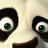## Le Monde puzzle [#929]A combinatorics Le Monde mathematical puzzle:

In the set {1,…,12}, numbers adjacent to i are called friends of i. How many distinct subsets of size 5 can be chosen under the constraint that each number in the subset has at least a friend with him?

In a brute force approach, I tried a quintuple loop to check all possible cases:

case=0
for (a in 1:(12-4))
for (b in (a+1):(12-3))
for (c in (b+1):(12-2))
for (d in (c+1):(12-1))
for (e in (d+1):12)
case=case+((b-a<2)&(min(c-b,d-c)<2)
&(min(d-c,e-d)<2)&(e-d<2))


which returns 64 possible cases. Note that the second and last loop are useless since b=a+1 and e=d+1, necessarily. And c is either (b+1) or (d-1), which means 2 choices for c, except when e=a+4. This all adds up to$8 + 2\sum_{a=1}^7\sum_{e=a+5}^{12} = 8+2.7.8-2.7.8/2=8.8=64$

A related R question: is there a generic way of programming a sequence of embedded loops like the one above without listing all of the loops one by one?

### 10 Responses to “Le Monde puzzle [#929]”

1.Jonathan Says:

#using Knuth’s algorithm for generating combinations
nextComb <- function(x,m){
ret <- x
l <- length(x)
if (x == m-l+1){
#anything to signify that we're done
return(rep(m,l))
}
else{
j<- match(FALSE,x==x+0:(l-1))
if(is.na(j)){j2 <- l} else{j2 <- j-1}
ret[j2] 1){ret[1:(j2-1)] <- 1:(j2-1)}
return(ret)
}

}
xx <- 1:5
count <- 0
while(xx<12){
if( all((xx+1) %in% xx |(xx-1) %in% xx)){cat(xx,"\n")
count=count+1}
xx <- nextComb(xx,12)}
cat(count,"\n")

2.Carl Witthoft Says:

This puzzle appears to be the “Schoolgirl walking group” puzzle in a different cloak. If so, I believe the general case of groups of size k from a set of size N is unsolved (that is, how many different sets of groups can be formed while maintaining or not maintaining a “friend” in each set of groups)

3.S. Panda (@thuspandas) Says:

combn() can take a function as an argument so you don’t even need apply().

is.friendset <- function(set) {
if ((set-set<2) &
(min(set-set,set-set)<2) &
(min(set-set,set-set)<2) &
(set-set<2)) TRUE else FALSE
}
num_friendsets <- sum(combn(12,5,is.friendset))
•xi'an Says:

Very novel from my perspective, thanks!

•S. Panda (@thuspandas) Says:

Thanks. I’m wondering though… do we need that last test to determine if it’s a valid set? Seems to me we can do away with:

(set-set<2)

I tried a handful of cases and it didn’t seem to matter. I would think the prior test catches the situation of the 5th element being a friend of the fourth element, so we could reduce it even further to:

is.friendset <- function(set) {
if ((set-set<2) &
(min(set-set,set-set)<2) &
(min(set-set,set-set)<2))
TRUE else FALSE
}
num_friendsets <- sum(combn(12,5,is.friendset))

I’m also wondering if there isn’t a more efficient way of calculating the result since if you increase the number of elements you’re drawing from, the execution time scales exponentially along with the number combinations and valid sets!

•xi'an Says:

Both the first and the last terms are defined by the values of the second and second-to-last, respectively. Hence the later values are free, kind of, and this leaves only the third to be constrained. For more than 5 terms, I do not know how this extends, though.

4.yatzek Says:

# for-loop-less approach

numberOfSlots <- 12
groupSize <- 5

# create new groups that include all numbers
# between smallest and largest elements
# that we started with
possibleGroupSizes <- seq(groupSize, numberOfSlots)

# number of distinct new groups of each size
numberOfArragements <- numberOfSlots + 1 – possibleSizes

# 5 is quite special so that …
numberOfArragements[-1] <- numberOfArragements[-1]*2

# and the final answer is …
sum(numberOfArragements)

•S. Panda (@thuspandas) Says:

Oh! Neato. I’m not sure why that works, but that’s a cool solution. Am I right in thinking that won’t work for groupSize 5?

5.Martyn Says:

Instead of nested loops you could use recursive function calls. It’s difficult to describe in more detail without giving example code.

However, in R it is usually simplest to work in terms of whole objects. If you load the combinat package then combn(12, 5) returns all distinct subsets in a matrix. Then you can use apply with a custom function to find which columns of the matrix satisfy the friend criterion.

•xi'an Says:

Yes, I knew of R combinat and should have used it..!

This site uses Akismet to reduce spam. Learn how your comment data is processed.